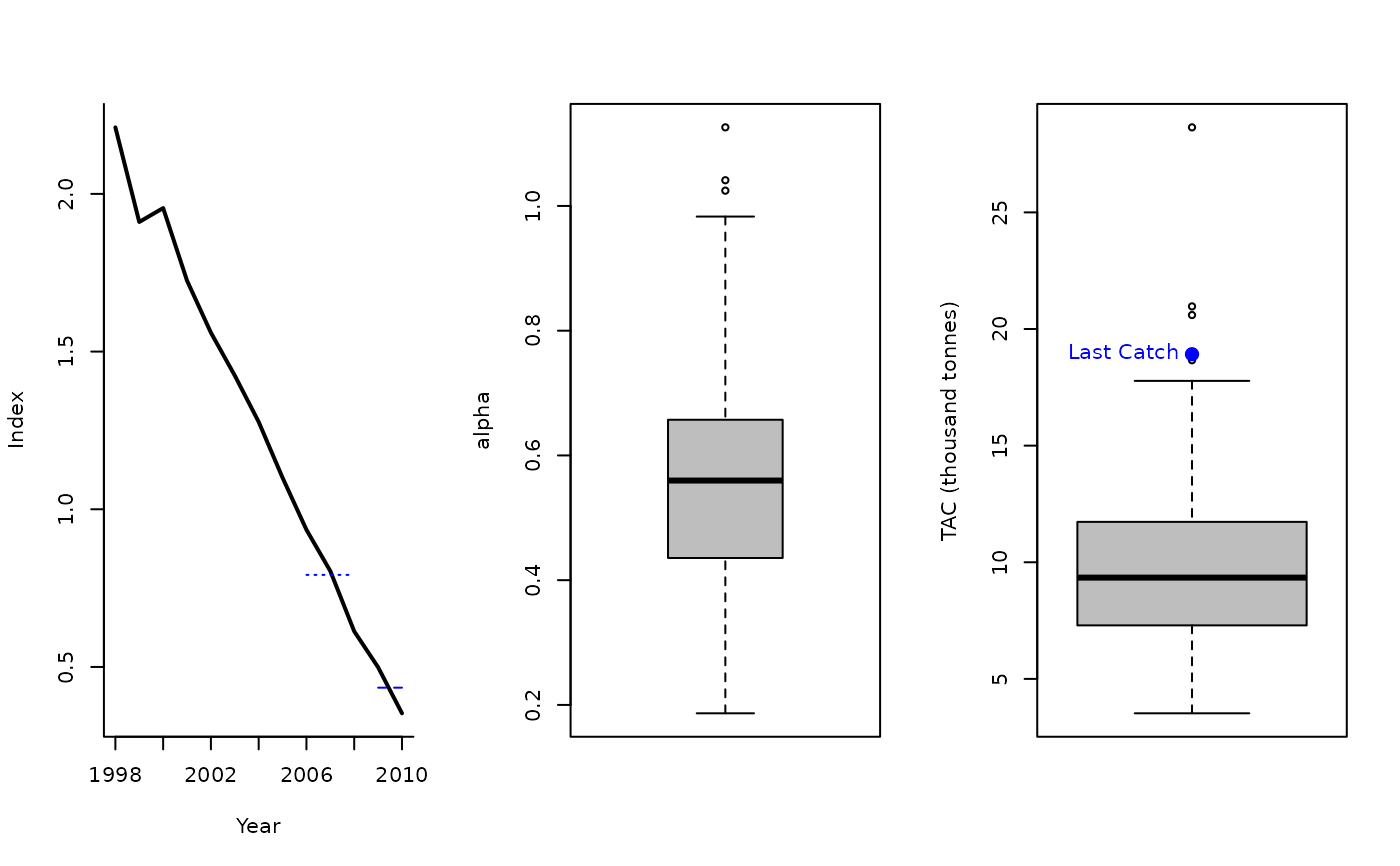The TAC is adjusted by the ratio alpha, where the numerator being the mean index in the most recent two years of the time series and the denominator being the mean index in the three years prior to those in the numerator. This MP is the stochastic version of Method 3.2 used by ICES for Data-Limited Stocks (ICES 2012).

Iratio(x, Data, reps = 100, plot = FALSE, yrs = c(2, 5))

## Arguments

x

A position in the data object

Data

A data object

reps

The number of stochastic samples of the MP recommendation(s)

plot

Logical. Show the plot?

yrs

Vector of length 2 specifying the reference years

## Value

An object of class Rec-class with the TAC slot populated with a numeric vector of length reps

## Details

The TAC is calculated as: $$\textrm{TAC}_y = \alpha C_{y-1}$$ where $$C_{y-1}$$ is the catch from the previous year, and $$\alpha$$ is the ratio of the mean index in the most recent two years of the time series and the mean index in 3-5 years before current time (reference years are specified as yrs argument.

## Required Data

See Data-class for information on the Data object

Iratio: Cat, Ind

## Rendered Equations

See Online Documentation for correctly rendered equations

Other Index methods: GB_slope(), GB_target(), Gcontrol(), ICI(), Islope1(), Itarget1_MPA(), Itarget1(), ItargetE1()

## Author

Coded by Q. Huynh. Developed by Jardim et al. (2015)

## Examples

Iratio(1, MSEtool::Atlantic_mackerel, plot=TRUE)#> TAC (median)
#>     9.706986